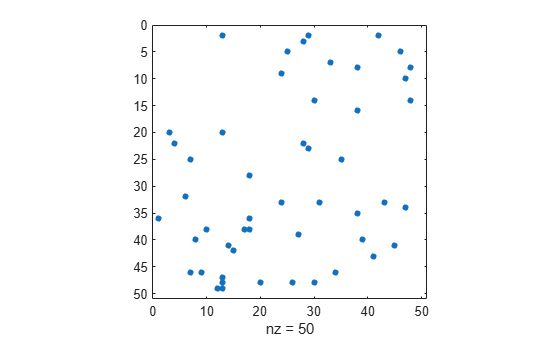# spfun

Apply function to nonzero sparse matrix elements

## Syntax

``F = spfun(func,S)``

## Description

example

````F = spfun(func,S)` applies the function `func` to the nonzero elements of a sparse matrix `S`. The input argument `func` is a function handle to a function that takes one input argument.This operation preserves the sparsity of the original matrix `S` unless the function `func` returns zero for some nonzero elements of `S`.```

## Examples

collapse all

Create a 4-by-4 sparse diagonal matrix.

`S = diag(sparse(1:4))`
```S = (1,1) 1 (2,2) 2 (3,3) 3 (4,4) 4 ```

Apply the exponential function to the nonzero elements of `S`. The resulting matrix has the same sparsity pattern as `S`.

`F = spfun(@exp,S)`
```F = (1,1) 2.7183 (2,2) 7.3891 (3,3) 20.0855 (4,4) 54.5982 ```

Because `spfun` only applies to nonzero elements of `S`, the value of `F(i)` is zero whenever `S(i)` is zero. This is different from applying the function to all elements of `S`. For example, compare the result to applying the exponential function to all elements of `S`. The `exp(S)` function returns `1` for the elements of `S` that are `0`s.

`full(exp(S))`
```ans = 4×4 2.7183 1.0000 1.0000 1.0000 1.0000 7.3891 1.0000 1.0000 1.0000 1.0000 20.0855 1.0000 1.0000 1.0000 1.0000 54.5982 ```

Create a random `50`-by-`50` sparse matrix with density `0.02`, where the matrix has `50` nonzero elements. Plot the sparsity pattern of the matrix `S`.

```rng default; S = sprand(50,50,0.02); spy(S)```Evaluate the quadratic function ${x}^{2}+x+1$ to the nonzero elements of `S`. The evaluated function using `spfun` has the same sparsity pattern as the matrix `S`.

```fun = @(x) x.^2 + x + 1; F = spfun(fun,S); spy(F)```## Input Arguments

collapse all

Input matrix. This matrix is typically (but not necessarily) sparse.

If `S` is a full matrix, then `F = spfun(func,S)` applies the function `func` to the nonzero elements of `S` and returns `F` as a sparse matrix.

Data Types: `double` | `logical`
Complex Number Support: Yes

Function to apply to the elements of the input array, specified as a function handle. The function should operate on `S` element-wise. For more information about function handles, see Create Function Handle.

Example: `@(n) n+1`

## Tips

• If `func` returns zero for inputs that are zero, you can use `func(S)` to return the same results as calling `spfun` on a sparse matrix `S`.

## Version History

Introduced before R2006a